Next: Uniform Flow Up: Axisymmetric Incompressible Inviscid Flow Previous: Axisymmetric Velocity Fields

# Axisymmetric Irrotational Flow in Spherical Coordinates

In an irrotational flow pattern, we can automatically satisfy the constraint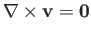by writing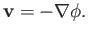(7.11)

Suppose, however, that, in addition to being irrotational, the flow pattern is also incompressible: that is,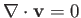. In this case, Equation (7.11) yields(7.12)

In spherical coordinates, assuming that the flow pattern is axisymmetric, so that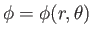, the previous equation leads to (see Section C.4)(7.13)

Let us search for a separable solution of Equation (7.13) of the form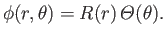(7.14)

It is easily seen that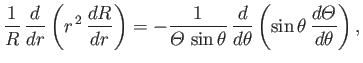(7.15)

which can only be satisfied provided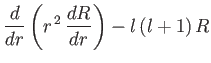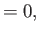(7.16)(7.17)

where, and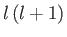is a constant. The solutions to Equation (7.17) that are well behaved for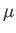in the range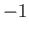toare known as the Legendre polynomials, and are denoted the), whereis a non-negative integer (Jackson 1962). (Ifis non-integer then the solutions are singular at) In fact,(7.18)

Hence,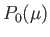(7.19)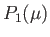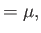(7.20)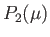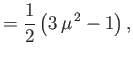(7.21)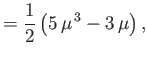(7.22)

et cetera. The general solution of Equation (7.16) is a linear combination ofand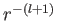factors. Thus, the general axisymmetric solution of Equation (7.12) is written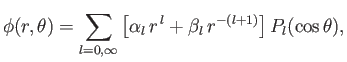(7.23)

where the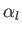and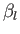are arbitrary coefficients. It follows from Equations (7.4) that the corresponding expression for the Stokes stream function is(7.24)

where.Next: Uniform Flow Up: Axisymmetric Incompressible Inviscid Flow Previous: Axisymmetric Velocity Fields
Richard Fitzpatrick 2016-03-31